Next: Index Up: Numbers Previous: A. Hints and Answers   Index

# B. Associativity and Distributivity of Operations in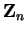Let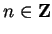satisfy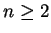. Let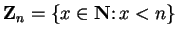. Let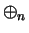and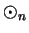be the binary operations ondefined by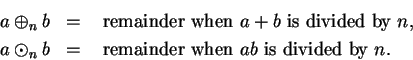Thus for all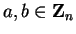,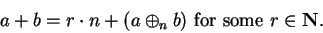(B.1)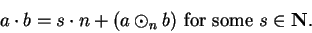(B.2)

We will show thatandare associative by using the usual properties of addition and multiplication on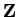.

B.3   Lemma. Let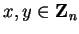,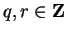. If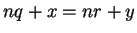, then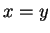and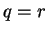.

Proof:

Case 1. Suppose. Then by our assumptions,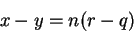and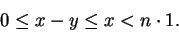So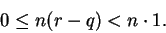Since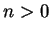, it follows that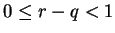and since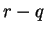is an integer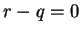, so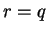. Then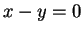, so.
Case 2. If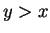, use Case 1 with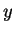and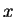interchanged.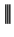B.4   Theorem.is associative on.

Proof: Let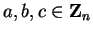. Then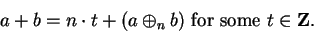(B.5)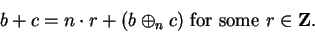(B.6)(B.7)(B.8)

By adding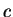to both sides of (B.5), we get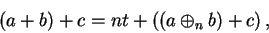(B.9)

and by adding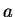to both sides of (B.6), we get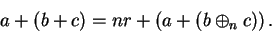(B.10)

Replace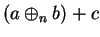in (B.9) by its value from (B.7) to get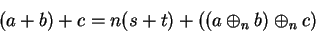(B.11)

and replace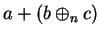in (B.10) by its value from (B.8) to get(B.12)

By (B.11) and (B.12) and the associative law in,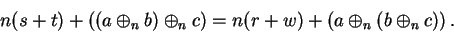the associativity offollows from lemma (B.3).B.13   Theorem.is associative on.

Proof: The proof is nearly identical with the proof thatis associative.

B.14   Theorem. The distributive law holds in; i.e., for all,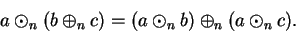Proof: We have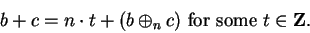(B.15)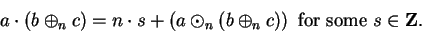(B.16)(B.17)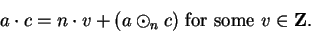(B.18)

Multiply both sides of (B.15) byto get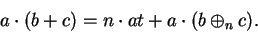(B.19)

Replace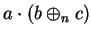in (B.19) by its value from (B.16) to get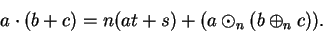(B.20)

Now add equations (B.17) and (B.18) to get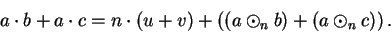(B.21)

We know that for some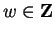,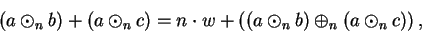and if we substitute this into (B.21), we obtain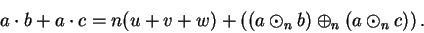(B.22)

From (B.20) and (B.22) and the distributive law in, we conclude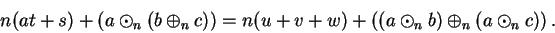The distributive law follows from lemma B.3.Next: Index Up: Numbers Previous: A. Hints and Answers   Index